This is “Introduction to Factoring”, section 6.1 from the book Beginning Algebra (v. 1.0). For details on it (including licensing), click here.

Has this book helped you? Consider passing it on:
Creative Commons supports free culture from music to education. Their licenses helped make this book available to you.
DonorsChoose.org helps people like you help teachers fund their classroom projects, from art supplies to books to calculators.

## 6.1 Introduction to Factoring

### Learning Objectives

1. Determine the greatest common factor (GCF) of natural numbers.
2. Determine the GCF of monomials.
3. Factor out the GCF of a polynomial.
4. Factor a four-term polynomial by grouping.

## GCF of Natural Numbers

The process of writing a number or expression as a product is called factoringThe process of writing a number or expression as a product.. If we write 60 = 5 ⋅ 12, we say that the product 5 ⋅ 12 is a factorizationAny combination of factors, multiplied together, resulting in the product. of 60 and that 5 and 12 are factorsAny of the numbers or expressions that form a product.. Typically, there are many ways to factor a number. For example,Recall that a prime number is defined as a natural number with exactly two natural number factors, 1 and itself. The first ten prime numbers follow:Any natural number greater than 1 can be uniquely written as a product of prime numbers. This product is called the prime factorizationThe unique factorization of a natural number written as a product of primes.. The prime factorization of 60 can be determined by continuing to factor until only a product of prime numbers remains.Since the prime factorization is unique, it does not matter how we choose to initially factor the number; the end result will be the same. The prime factorization of 60 follows:Recall that the greatest common factor (GCF)The product of all the common prime factors. of any two natural numbers is the product of all the common prime factors.

Example 1: Find the GCF of 60 and 140.

Solution: First, determine the prime factorizations of both integers.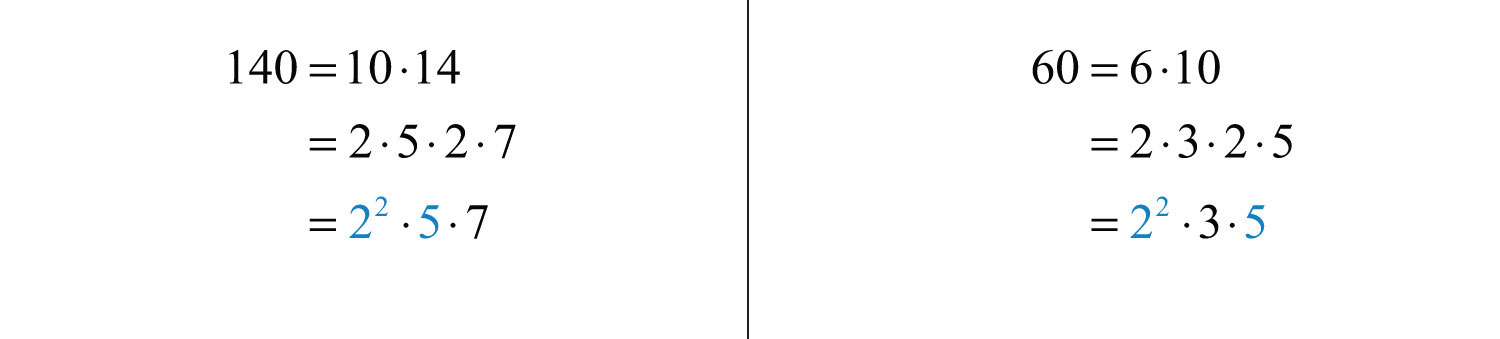The product of the common prime factors is $2 2 ⋅5$; hence the $GCF(60, 140)= 2 2⋅5=20$. To see that it is the greatest common factor, we can write the following:Answer: The greatest common factor of 60 and 140 is 20.

Example 2: Find the GCF of 504 and 1,080.

Solution: First, determine the prime factorizations of both integers.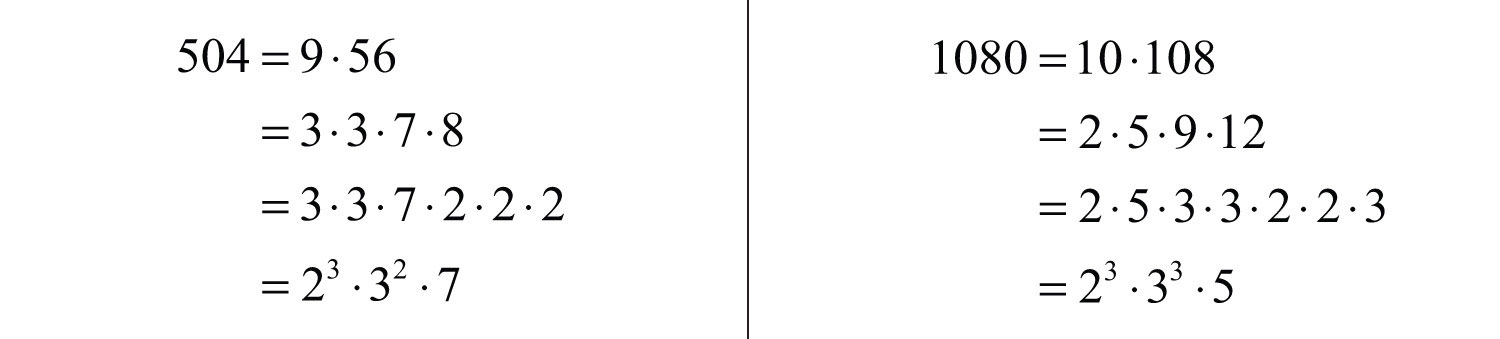The product of the common prime factors is $23⋅32$. The $GCF(504, 1080)=23⋅32=72$. Note that we multiplied the common prime factors with the smallest exponent.The numbers 7 and 15 share no common natural number factor other than 1; we say that they are relatively primeExpressions that share no common factors other than 1..

Answer: The greatest common factor of 504 and 1,080 is 72.

## GCF of Monomials

We next consider factorizations of monomials. For example, $6x$ and $x4$ are factors of $6x5$ because $6x5=6x⋅x4$. Typically, there are many ways to factor a monomial. Some factorizations of $6x5$ follow:Given two or more monomials, it will be useful to find the greatest common monomial factor of each. For example, consider $6x5y3z$ and $8x2y3z2$. The variable part of these two monomials look very much like the prime factorization of natural numbers and, in fact, can be treated the same way. Steps for finding the GCF of monomialsThe product of the GCF of the coefficients and all common variable factors. are outlined in the following example.

Example 3: Find the GCF of $6x5y3z$ and $8x2y3z2$.

Solution:

Step 1: Find the GCF of the coefficients.In this case, the $GCF(6, 8)=2$.

Step 2: Determine the common variable factors with smallest exponents.In this case, the common variables with the smallest exponents are $x2$, $y3$, and $z1$.

Step 3: The GCF of the monomials is the product of the common variable factors and the GCF of the coefficients. Therefore,Answer: $2x2y3z$

It is worth pointing out that the GCF in the previous example divides both expressions evenly:Furthermore, we can write the following:The factors $3x3$ and $4z$ share no common monomial factors other than 1; they are relatively prime.

Example 4: Determine the GCF of the following expressions: $30x6y$ and $18x4y2z$.

Solution: The prime factorizations of the coefficients areThus the GCF(30, 18) = 2 ⋅ 3 = 6. Next, consider the variable part:The variable factors in common are $x4$ and y. The factor z is not in common and we haveAnswer: $6x4y$

Example 5: Determine the GCF of the following three expressions: $12a5b2(a+b)5$, $60a4b3c(a+b)3$, and $24a2b7c3(a+b)2$.

Solution: First, determine the GCF of the coefficients.The . Next, determine the common factors of the variable part: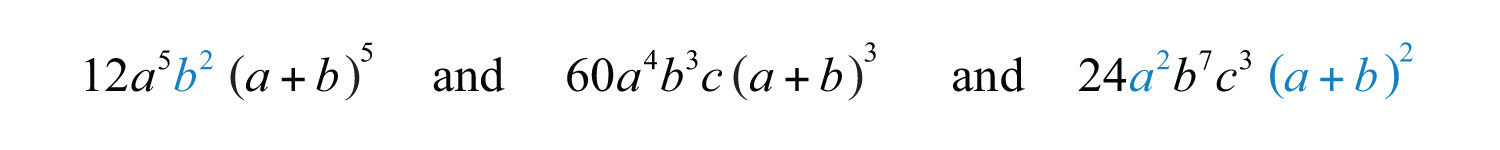The variable factors in common are $a2$, $b2$, and $(a+b)2$. Therefore,Answer: $12a2b2(a+b)2$. Note that the variable c is not common to all three expressions and thus is not included in the GCF.

Try this! Determine the GCF of the following: $60x4y3(x+2y)7$, $45x2y5(x+2y)4$, and $30x7y7(x+2y)3$.

Answer: $15x2y3(x+2y)3$

### Video Solution

(click to see video)

## Factoring out the GCF

We have seen that application of the distributive property is the key to multiplying polynomials. The process of factoring a polynomialThe process of rewriting a polynomial as a product of polynomial factors. involves using the distributive property in reverse to write each polynomial as a product of polynomial factors.To demonstrate this idea, we multiply and factor side by side. Factoring utilizes the GCF of the terms.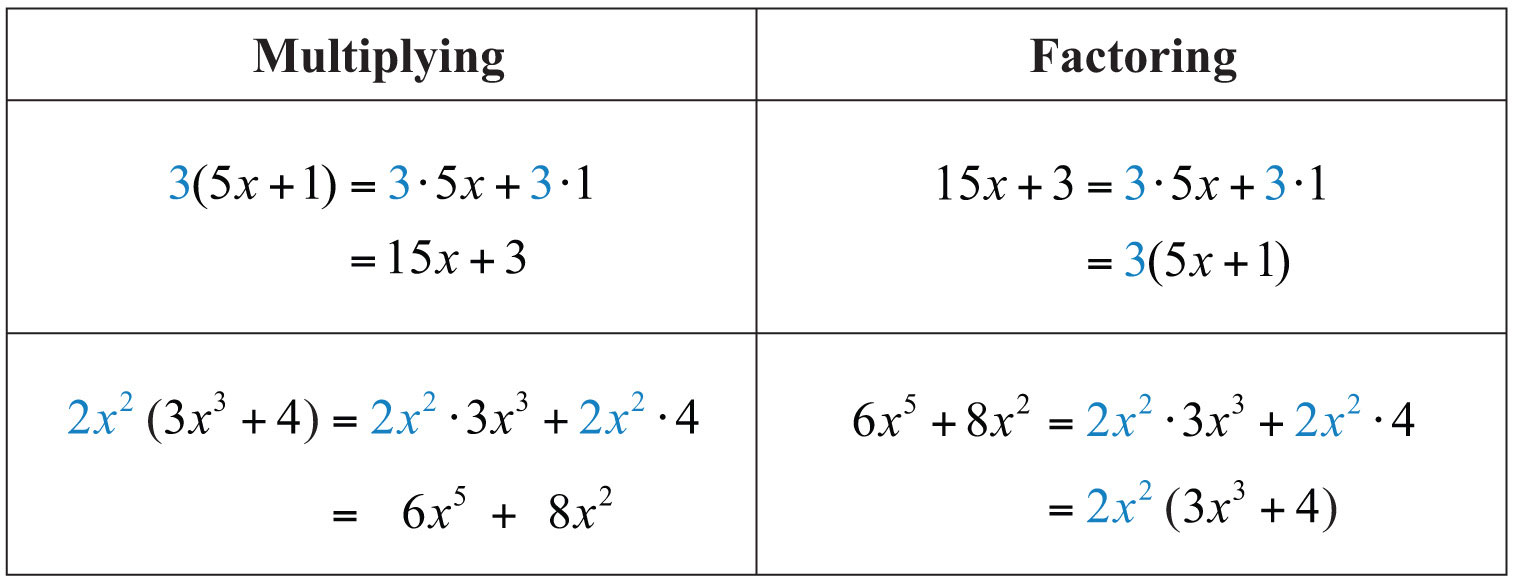In the previous example, we see that the distributive property allows us to write the polynomial $6x5+8x2$ as a product of the two factors $2x2$ and $(3x3+4)$. Note that in this case, $2x2$ is the GCF of the terms of the polynomial:Factoring out the GCFThe process of rewriting a polynomial as a product using the GCF of all of its terms. involves rewriting a polynomial as a product where a factor is the GCF of all of its terms:The steps for factoring out the GCF of a polynomialThe greatest common factor of all the terms of the polynomial. are outlined in the following example.

Example 6: Factor out the GCF: $7x4+21x3−14x2$.

Solution:

Step 1: Identify the GCF of all the terms. In this case, the GCF(7, 21, 14) = 7, and the common variable factor with the smallest exponent is $x2$. The GCF of the polynomial is $7x2$.Step 2: Determine the terms of the missing factor by dividing each term of the original expression by the GCF. (This step is usually performed mentally.)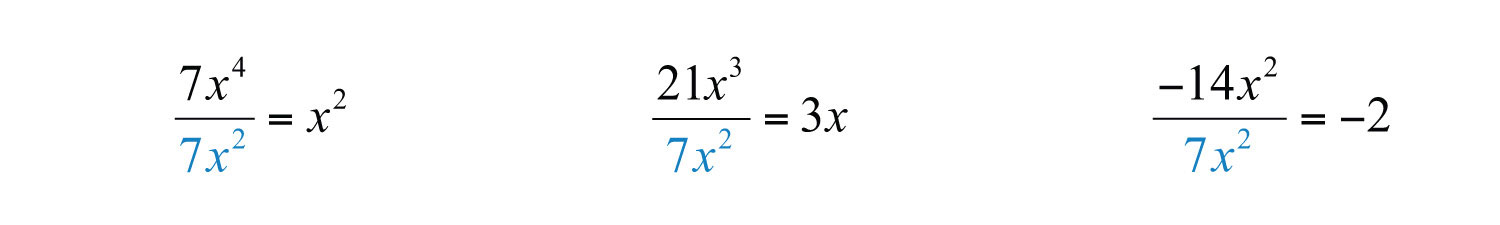Step 3: Apply the distributive property (in reverse) using the terms found in the previous step.Step 4: As a check, multiply using the distributive property to verify that the product equals the original expression. (This step is optional and can be performed mentally.)Answer: $7x2(x2+3x−2)$

Example 7: Factor out the GCF: $48a−16b+4c$.

Solution: There are no variable factors in common and the GCF(48, 16, 4) = 4.Answer: $4(12a−4b+c)$

Example 8: Factor out the GCF: $25x3+15x2+5x$.

Solution: The GCF(25, 15, 5) = 5, and the common variable factor with smallest exponents is $x1$. The GCF of all the terms is $5x$.Answer: $5x(5x2+3x+1)$

If the GCF is the same as one of the terms, then, after the GCF is factored out, a constant term 1 will remain. In the previous example, we can see that $5x5x=1$. The importance of remembering the constant term becomes clear when performing the check using the distributive property:The constant term 1 allows us to obtain the same original expression after we distribute.

Example 9: Factor out the GCF: $15x6y4+10x5y3z2−20xy6z3$.

Solution: The $GCF(10, 15, 20)=5$, and the common variables with smallest exponent are $x1$ and $y3$. Therefore, the GCF of the terms is $5xy3$. The first term does not have a variable factor of $z$ and thus cannot be a part of the greatest common factor. If we divide each term by $5xy3$, we obtain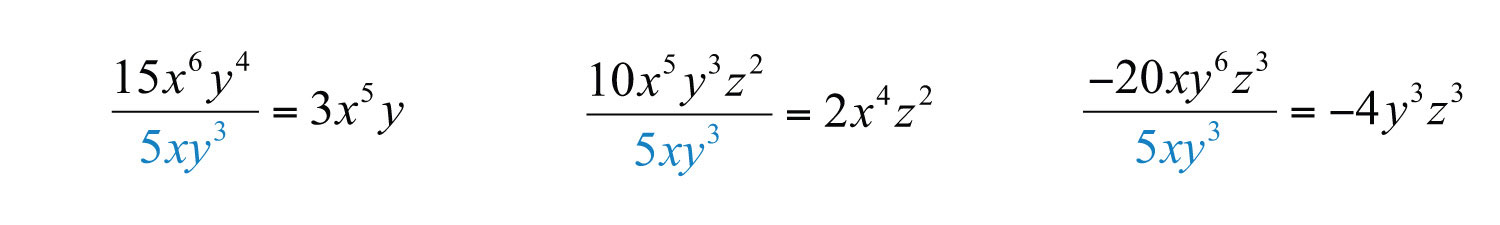and can write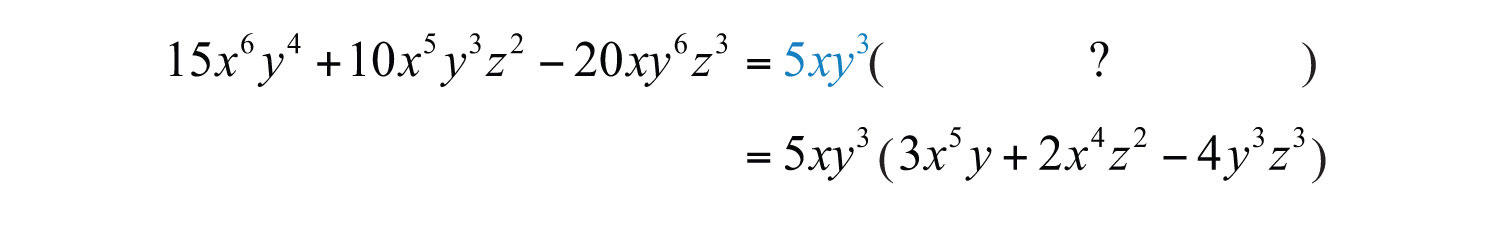Answer: $5xy3(3x5y+2x4z2−4y3z3)$

Example 10: Factor out the GCF: $24a6b2c5+8a7b5c$.

Solution: The GCF(24, 8) = 8, and the variable factors with smallest exponents are $a6$, $b2$, and $c$. Therefore, the GCF of all the terms is $8a6b2c$.Answer: $8a6b2c(3c4+ab3)$

Of course, not all polynomials with integer coefficients can be factored as a product of polynomials with integer coefficients other than 1 and itself. If this is the case, then we say that it is a prime polynomialA polynomial with integer coefficients that cannot be factored as a product of polynomials with integer coefficients other than 1 and itself..

Example 11: Factor: $3x−5$.

Solution: Prime: there are no polynomial factors other than 1 and itself.

Try this! Factor out the GCF: $16x4y3−8x2y5−4x2y$.

Answer: $4x2y(4x2y2−2y4−1)$

### Video Solution

(click to see video)

## Factor by Grouping

In this section, we outline a technique for factoring polynomials with four terms. First, review some preliminary examples where the terms have a common binomial factor.

Example 12: Factor: $5x(x−3)+2(x−3)$.

Solution: This expression is a binomial with terms $5x(x−3)$ and $2(x−3)$. In this case, $(x−3)$ is a common factor. Begin by factoring this common factor out:To determine the terms of the remaining factor, divide each term by $(x−3)$:This step is typically performed mentally. We haveAnswer: $(x−3)(5x+2)$

Recall that 1 is always a common factor. If the GCF is the same as a term, then the factor 1 remains after we factor out that GCF.

Example 13: Factor: $3x(4x+1)−(4x+1)$.

Solution: Rewrite the second term $−(4x+1)$ as $−1(4x+1)$ and then factor out the common binomial factor $(4x+1)$.Answer: $(4x+1)(3x−1)$

Remember that the goal for this section is to develop a technique that enables us to factor polynomials with four terms into a product of binomials. The intermediate step of this process looks like the previous two examples. For example, we wish to factorBegin by grouping the first two terms and the last two terms. Then factor out the GCF of each grouping:In this form, it is a binomial with a common binomial factor, $(x−3)$.The steps that follow outline a technique for factoring four-term polynomials called factor by groupingA technique for factoring polynomials with four terms..

Example 14: Factor: $2x3+4x2+3x+6$.

Solution: Group terms in such a way as to obtain a binomial with common factors.

Step 1: Group the first two and last two terms and then factor out the GCF of each.The GCF of the first two terms is $2x2$, and the GCF of the second two terms is 3.Step 2: At this point, the polynomial is a binomial. Factor out any factors common to both terms. Here $(x+2)$ is a common factor.Step 3: Optional check: multiply to verify that we obtain the original expression.Answer: $(x+2)(2x2+3)$

Example 15: Factor: $2a3−3a2+2a−3$.

Solution: The GCF of the first two terms is $a2$ and the GCF of the second two terms is 1.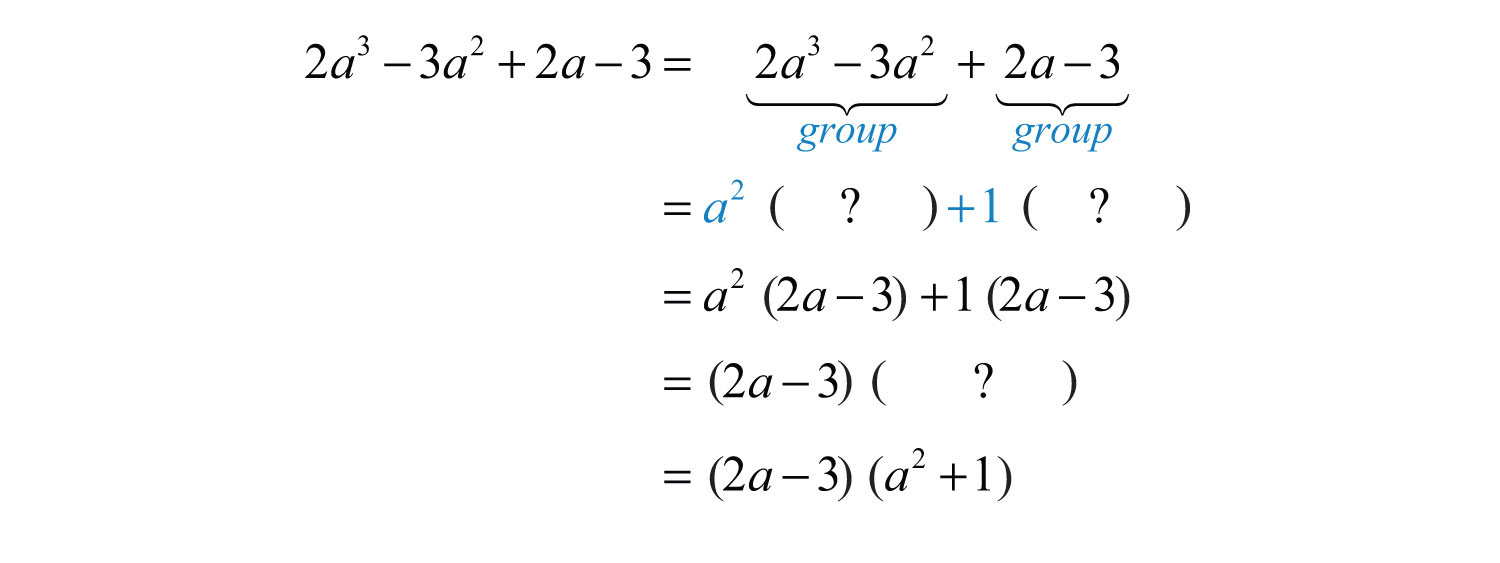Answer: $(2a−3)(a2+1)$. The check is left to the reader.

Example 16: Factor: $6x4−24x3−5x+20$.

Solution: The GCF for the first group is $6x3$. We have to choose 5 or −5 to factor out of the second group.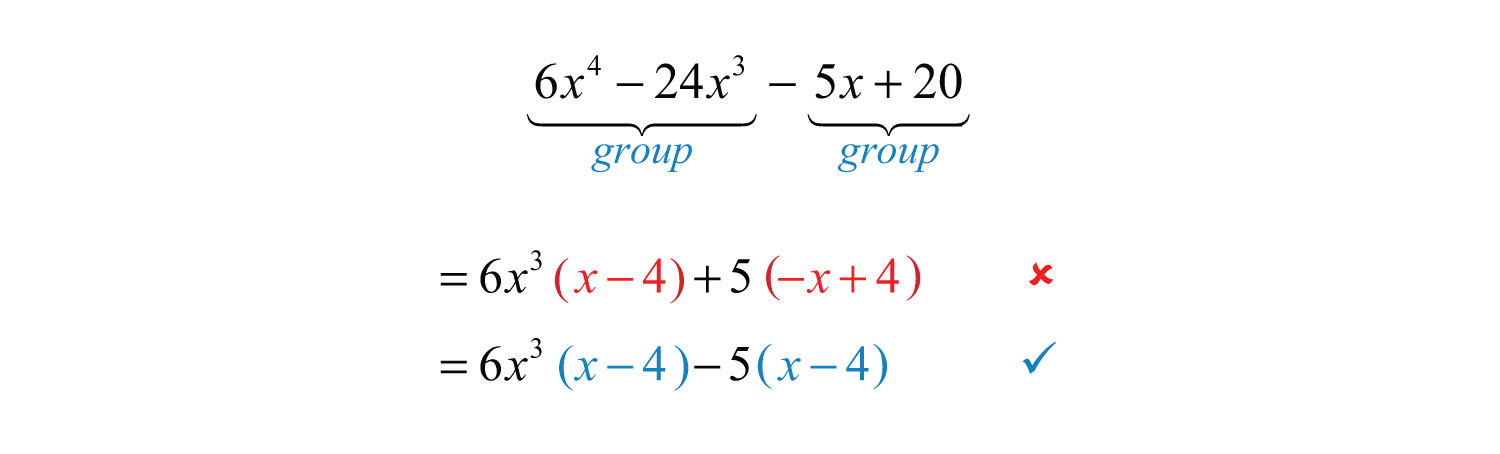Factoring out a +5 does not result in a common binomial factor. If we choose to factor out −5, then we obtain a common binomial factor and can proceed. Note that when factoring out a negative number, we change the signs of the factored terms.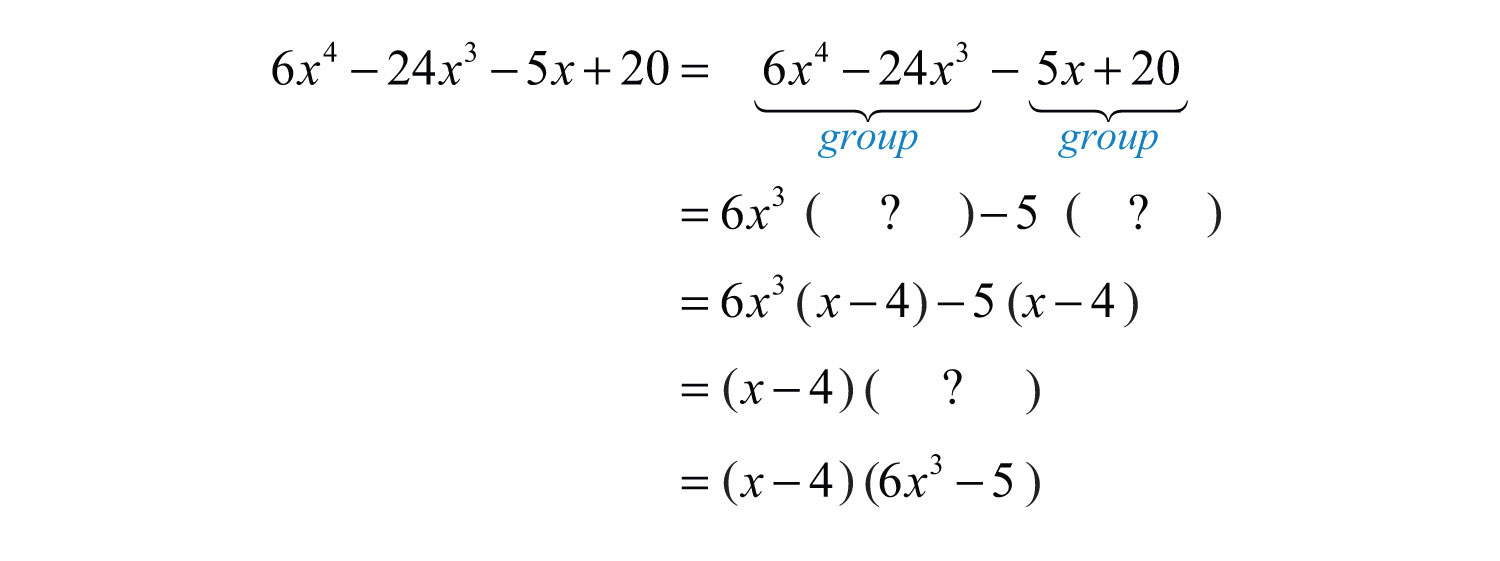Answer: $(x−4)(6x3−5)$. The check is left to the reader.

### Tip

The sign of the leading coefficient in the second grouping usually indicates whether or not to factor out a negative factor. If that coefficient is positive, factor out a positive factor. If it is negative, factor out a negative factor.

When all the terms of a polynomial have a GCF other than 1, it is a best practice to factor that out before factoring by grouping.

Example 17: Factor: $3y4+9y2−6y3−18y$.

Solution: Here we notice that the greatest common factor of all the terms is $3y$. Begin by factoring out the GCF and then factor the result by grouping.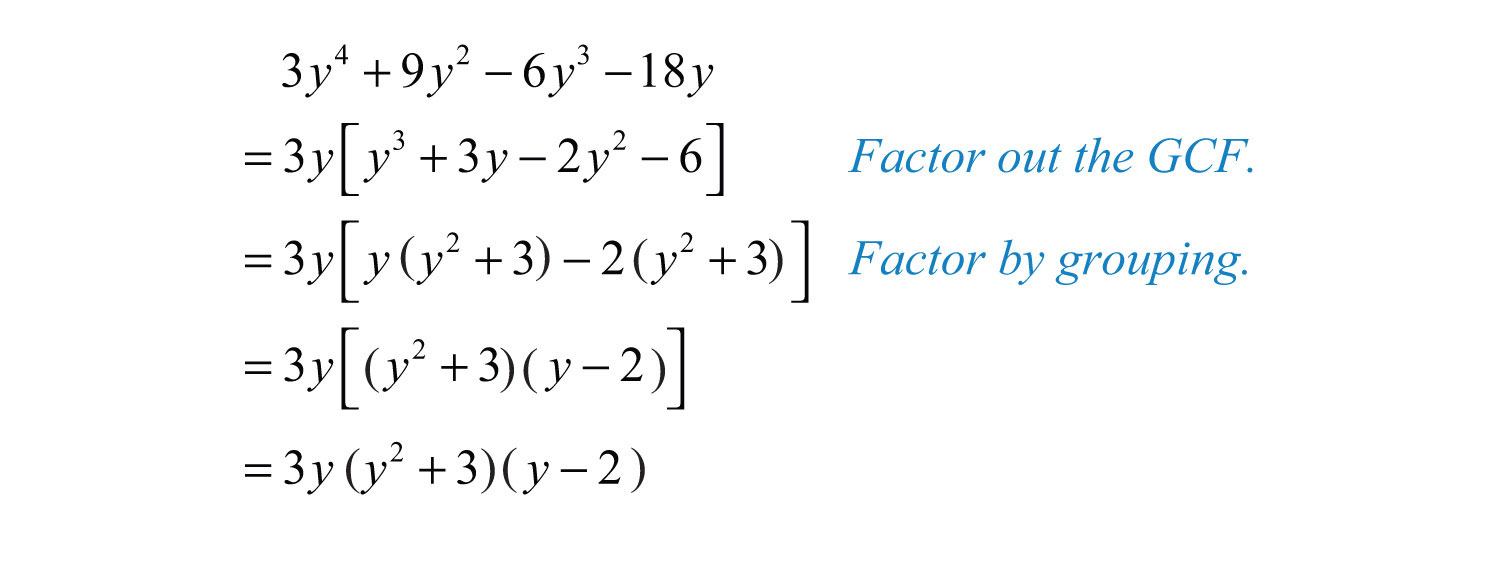Answer: $3y(y2+3)(y−2)$

Sometimes we must first rearrange the terms in order to obtain a common factor.

Example 18: Factor: $ab−2a2b+a3−2b3$.

Solution: Simply factoring the GCF out of the first group and last group does not yield a common binomial factor.We must rearrange the terms, searching for a grouping that produces a common factor. In this example, we have a workable grouping if we switch the terms $a3$ and $ab$.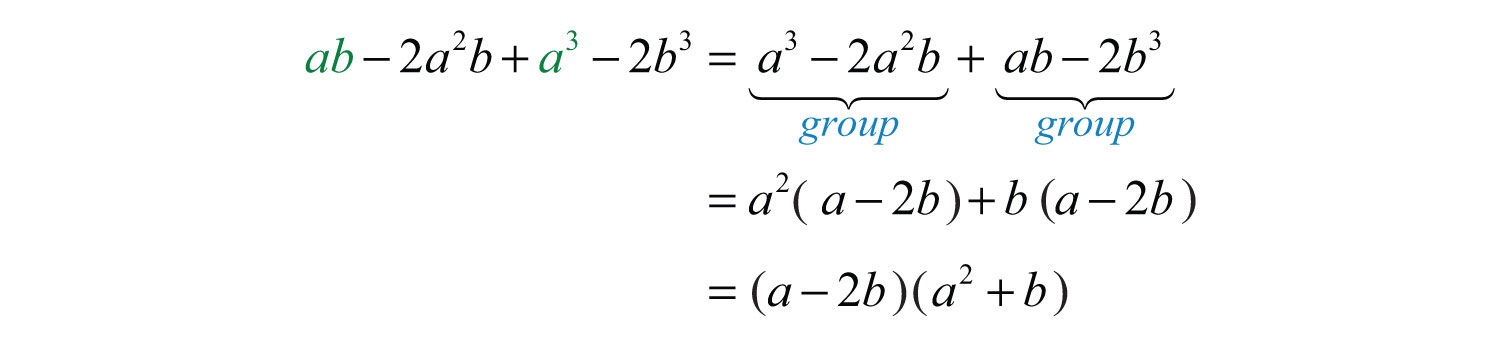Answer: $(a−2b)(a2+b)$

Not all factorable four-term polynomials can be factored with this technique. For example,This four-term polynomial cannot be grouped in any way as to produce a common binomial factor. Despite this, the polynomial is not prime and can be written as a product of polynomials. It can be factored as follows:Factoring such polynomials is something that we will learn to do as we move further along in our study of algebra. For now, we will limit our attempt to factor four-term polynomials to using the factor by grouping technique.

Try this! Factor: $x3−x2y−xy+y2$.

Answer: $(x−y)(x2−y)$

### Video Solution

(click to see video)

### Key Takeaways

• To find the greatest common factor (GCF) of any collection of natural numbers, first find the prime factorization of each. The GCF is the product of all the common prime factors.
• The GCF of two or more monomials is the product of the GCF of the coefficients and the common variable factors with the smallest power.
• If the terms of a polynomial have a greatest common factor, then factor out that GCF using the distributive property. Divide each term of the polynomial by the GCF to determine the terms of the remaining factor.
• Some four-term polynomials can be factored by grouping the first two terms and the last two terms. Factor out the GCF of each group and then factor out the common binomial factor.
• When factoring by grouping, you sometimes have to rearrange the terms to find a common binomial factor. After factoring out the GCF, the remaining binomial factors must be the same for the technique to work.
• Not all polynomials can be factored as the product of polynomials with integer coefficients. In this case, we call it a prime polynomial.

### Topic Exercises

Part A: GCF of Natural Numbers

Give the prime factorization of each number and determine the GCF.

1. 18, 24

2. 45, 75

3. 72, 60

4. 168, 175

5. 144, 245

6. 15, 50, 60

7. 14, 63, 70

8. 12, 48, 125

9. 60, 72, 900

10. 252, 336, 360

Part B: GCF of Variable Expressions

Determine the GCF of all the terms.

11. $15x, 30$

12. $14x, 21$

13. $45x4, 8x3$

14. $36x5, 35y2$

15. $6x, 27x, 36x$

16. $12x3, 4x2, 6x$

17. $12x2y, 60xy3$

18. $7ab2, 2a2b, 3a3b3$

19. $6a2b2, 18a3b2, 9ab2$

20. $15x(x+2), 9(x+2)$

21. $20x(2x−1), 16(2x−1)$

22. $20x3(x+y)5, 10x5(x+y)2$

Part C: Factoring out the GCF

Given the GCF, determine the missing factor.

23. $25x2+10x=5x( ? )$

24. $12y5+7y2=y2( ? )$

25. $22x4−121x2+11x=11x( ? )$

26. $30y3−45y2−3y=3y( ? )$

27. $36a5b7−60a6b5=12a5b5( ? )$

28. $24x2y+48xy2−12xy=12xy( ? )$

Factor out the GCF.

29. $4x−8$

30. $27x−9$

31. $3x−18$

32. $5x−10$

33. $25x−16$

34. $72x−35$

35. $15x2+30x$

36. $14a2−7a$

37. $30a5−10a2$

38. $8x4−16x2$

39. $5x6+x3$

40. $3x7−9x5$

41. $18a2+30a−6$

42. $24a2−36a−12$

43. $27x3−6x2+3x$

44. $8x3−12x2+2x$

45. $9x4+18x3−3x2$

46. $12y4−16y3+20y2$

47. $7x5−21x3−14x2+28x$

48. $36y10+12y8−18y4−6y3$

49. $12x5y2−8x3y$

50. $125a8b4c3−25a2b3c3$

51. $6x4y3−4x3y2+8x2y$

52. $15x4y2−30x3y3+15x2y4$

53. $81x7y6z2−18x2y8z4+9x2y5z2$

54. $4x5y4z9+26x5y3z4−14x6y8z5$

55. $2x(x−3)+5(x−3)$

56. $3x(2x+1)−4(2x+1)$

57. $5x(5x+2)−(5x+2)$

58. $2x(3x+4)+(3x+4)$

59. $x2(4x−7)−5(4x−7)$

60. $(x+6)−3x2(x+6)$

61. $(a+b)2−3a(a+b)2$

62. $(ab+2)3+3ab(ab+2)3$

63. $7x(x+7)5+14x2(x+7)5$

64. $36x5(3x+2)4−12x3(3x+2)4$

Are the following factored correctly? Check by multiplying.

65. $4x2−16x=4x(x−4)$

66. $3a3−3a=3a(a2)$

67. $3x3−5x6=x3(3−x2)$

68. $5x3−10x4+15x5=5x3(1−2x+3x2)$

69. $x3−x2+x=x(x2−x)$

70. $12x4y3−16x5y2+8x6y7=4x4y2(3y−4x+2x2y5)$

Use polynomial long division to show that the given factor divides the polynomial evenly.

71. Show that $(x−1)$ is a factor of $(2x3−5x2+4x−1)$.

72. Show that $(x+3)$ is a factor of $(3x3+7x2−4x+6)$.

73. Show that $(3x−2)$ is a factor of $(3x3+4x2−7x+2)$.

74. Show that $(2x+1)$ is a factor of $(2x3−5x2+x+2)$.

75. The height in feet of an object tossed into the air is given by the function $h(t)=−16t2+32t$, where t is the time in seconds after it is tossed. Write the function in factored form.

76. The height in feet of an object dropped from a 16‑foot ladder is given by the function $h(t)=−16t2+16$, where t is the time in seconds after it is tossed. Write the function in factored form.

77. The surface area of a cylinder is given by the formula $SA=2πr2+2πrh$, where r represents the radius of the base and h is the height of the cylinder. Express this formula in factored form.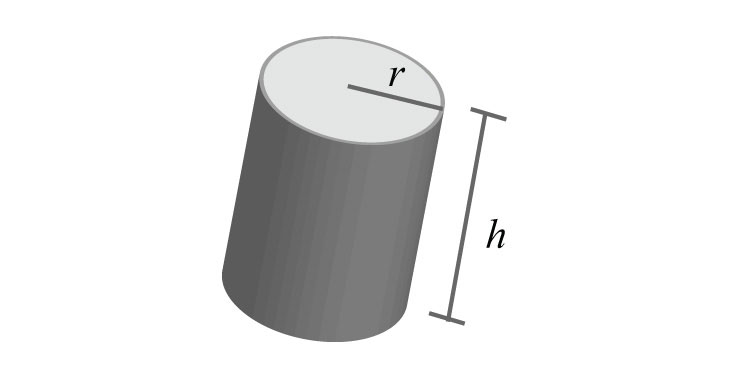78. The surface area of a cone is given by the formula $SA=πr2+πrs$, where r represents the radius of the base and s represents the slant height. Express this formula in factored form.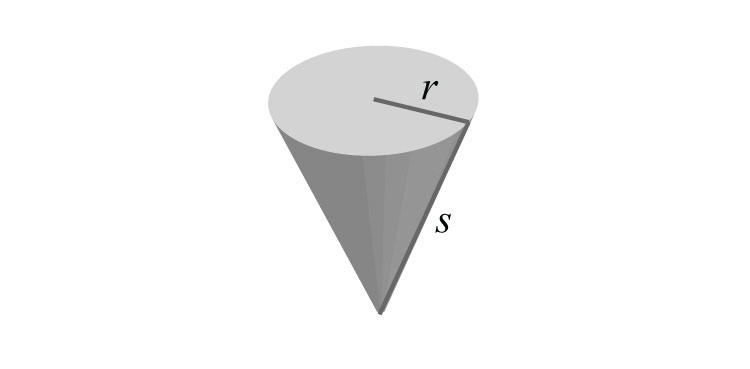Part D: Factor by Grouping

Factor by grouping.

79. $x2−10x+2x−20$

80. $x2−6x−3x+18$

81. $x3+2x2+2x+4$

82. $x3−3x2+5x−15$

83. $x3+7x2−2x−14$

84. $2x3+2x2−x−1$

85. $x3−5x2+4x−20$

86. $6x3−3x2+2x−1$

87. $9x3−6x2−3x+2$

88. $2x4−x3−6x+3$

89. $x5+x3+2x2+2$

90. $6x5−4x3−9x2+6$

91. $3a3b+3ab2+2a2+2b$

92. $2a3+2ab3−3a2b−3b4$

93. $2a3−a2b2−2ab+b3$

94. $a4−3a3b2+ab2−3b4$

95. $3a2b4−6b3−a2b+2$

96. $3x3+2y3+x3y3+6$

97. $−3x3−5y3+x3y3+15$

98. $2x3y3+2−y3−4x3$

99. $3x2−y3+xy2−3xy$

100. $2x2+y3−2xy−xy2$

Factor out the GCF first and then factor by grouping.

101. $5x2−35x−15x+105$

102. $12x2−30x−12x+30$

103. $2x3+6x2−10x−30$

104. $6x3−3x2−42x+21$

105. $4x4+4x3−12x2−12x$

106. $−9x4+6x3−45x2+30x$

107. $−12x5+4x4+6x3−2x2$

108. $24x5−36x4+8x3−12x2$

109. $24a3b2−60a3b+40ab2−100ab$

110. $a4b2−2a3b3+a2b3−2ab4$

Part E: Discussion Board Topics

111. Research the Euclidean algorithm for finding the GCF of two natural numbers. Give an example that illustrates the steps.

112. Research and discuss the contributions of Euclid of Alexandria.

113. Explain what factoring is and give an example.

114. Is $5x(x+2)−3(x+2)$ fully factored? Explain.

115. Make up a factoring problem of your own and provide the answer. Post the problem and the solution on the discussion board.

1: $18=2⋅32$, $24=23⋅3$, GCF = 6

3: $72=23⋅32$, $60=22⋅3⋅5$, GCF = 12

5: $144=24⋅32$, $245=5⋅72$, GCF = 1

7: $14=2⋅7$, $63=32⋅7$, $70=2⋅5⋅7$, GCF = 7

9: $60=22⋅3⋅5$, $72=23⋅32$, $900=22⋅32⋅52$, GCF = 12

11: 15

13: $x3$

15: $3x$

17: $12xy$

19: $3ab2$

21: $4(2x−1)$

23: $(5x+2)$

25: $(2x3−11x+1)$

27: $(3b2−5a)$

29: $4(x−2)$

31: $3(x−6)$

33: Prime

35: $15x(x+2)$

37: $10a2(3a3−1)$

39: $x3(5x3+1)$

41: $6(3a2+5a−1)$

43: $3x(9x2−2x+1)$

45: $3x2(3x2+6x−1)$

47:

49: $4x3y(3x2y−2)$

51: $2x2y(3x2y2−2xy+4)$

53:

55: $(x−3)(2x+5)$

57: $(5x+2)(5x−1)$

59: $(4x−7)(x2−5)$

61: $(a+b)2(1−3a)$

63: $7x(x+7)5(1+2x)$

65: Yes

67: No

69: No

75: $h(t)=−16t(t−2)$

77: $SA=2πr(r+h)$

79: $(x−10)(x+2)$

81: $(x+2)(x2+2)$

83: $(x+7)(x2−2)$

85: $(x−5)(x2+4)$

87: $(3x−2)(3x2−1)$

89: $(x2+1)(x3+2)$

91: $(a2+b)(3ab+2)$

93: $(a2−b)(2a−b2)$

95: $(3b3−1)(a2b−2)$

97: $(x3−5)(y3−3)$

99: $(x−y)(3x+y2)$

101: $5(x−7)(x−3)$

103: $2(x+3)(x2−5)$

105: $4x(x+1)(x2−3)$

107: $−2x2(3x−1)(2x2−1)$

109: $4ab(3a2+5)(2b−5)$